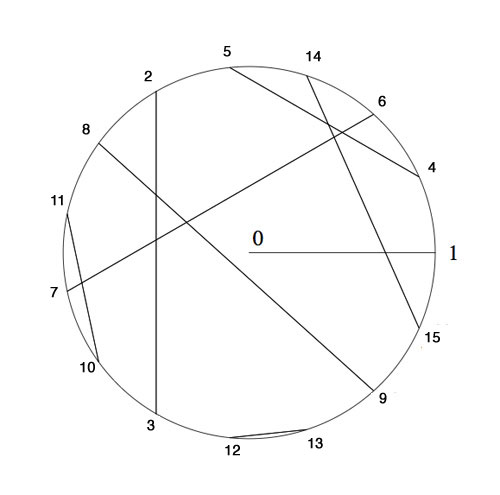Tag: Galois theory

The odd Knight of the round table problem asks for a consistent placement of the n-th Knight in the row at an odd root of unity, compatible with the two different realizations of the algebraic closure of the field with two elements.

The first identifies the multiplicative group of its non-zero elements with the group of all odd complex roots of unity, under complex multiplication. The second uses Conway’s ‘simplicity rules’ in ONAG to define an addition and multiplication on the set of all ordinal numbers.Here’s the seating arrangement for the first 15 knights. Conway proved that all finite ordinals smaller than $2^{2^i}$ form a subfield of $\overline{\mathbb{F}}_2$. The first non-trivial one being $\{ 0,1,2,3 \}$ with smallest multiplicative generator $2$, whence we place Knight 2 at $e^{2 i \pi/3}$ and as $2^2=3$ we know where to place the third Knight.

The next subfield is made of the numbers $\{ 0,1,2,\ldots,13,14,15 \}$ and its non-zero numbers form a cyclic group of order 15. Hence we need to find the smallest generator of this group satisfying the additional property of being compatible with the earlier seating, that is, its fifth power must equal to 2. Checking the multiplication table reproduced here one verifies that the wanted generator is 4 and so we place Knight 4 at $e^{\frac{2 \pi i}{15}}$ and as all the ordinals smaller than 16 are powers of 4 this tells us where to place the Knights until we get to the 15th in the row.

In february we were able to seat the first few thousand Knights by showing by hand that 32 is the smallest ordinal such that its 15-th power is equal to 4 and using SAGE that 1051 is the smallest ordinal whose 257-th power equals 32. These calculations enabled us to seat the Knights until we get to the 65536-th in the row.

Today I managed to show that 1361923 is the smallest ordinal such that its 65537-th power equals 1051. You can verify this statement in SAGE using the method explained in the february post

sage: R.< x,y,z,t,u >=GF(2)[]

sage: S.< a,b,c,d,e > =
R.quotient((x^2+x+1,y^2+y+x,z^2+z+x*y,t^2+t+x*y*z,u^2+u+x*y*z*t))

sage: (c*e+b*e+a*b*c*d+b*c*d+a*b*d+a+1)^65537
c^2 + b*d + a + 1

(It takes a bit longer to check minimality of 1361923). That is, we have to seat Knight 1361923 at $e^{\frac{2 \pi i}{4294967295}}$ and because all the numbers smaller than 4294967296 are powers of 1361923 we have seating arrangements for the first 4294967295 Knights!

I did try the same method in february but ran into time- and memory-problems on my 2.4Ghz 2Gb MacBook. Today I upgraded from Sage 3.3 to Sage 4.6 and this version is a lot faster (using the 64-bit architecture) and also appears to be much better at memory-management. Thank you, Sage-community!

Wishing you all a lot of mathematical (and other) fun in the prime-number year 2011.

atb :: lieven.